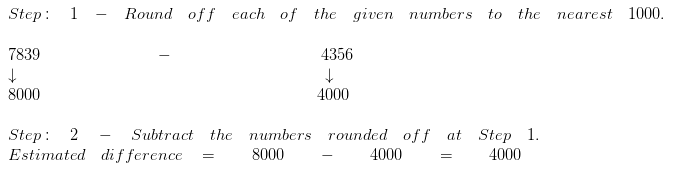# Estimate the sum and differences | Maths Grade 4

## Estimate the sum

Question 1

Find the estimated sum of 32 and 14?

Explanation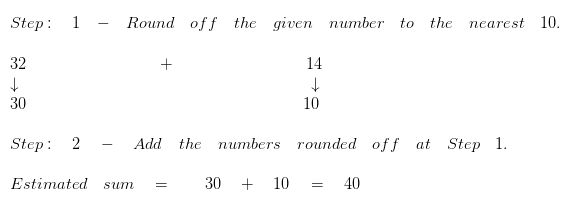Question 2

Find the estimated sum of 317 and 675?

Explanation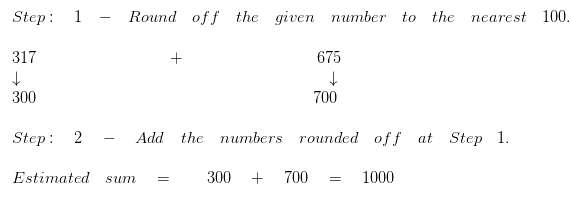Question 3

Find the estimated sum of 3783 and 6354?

Explanation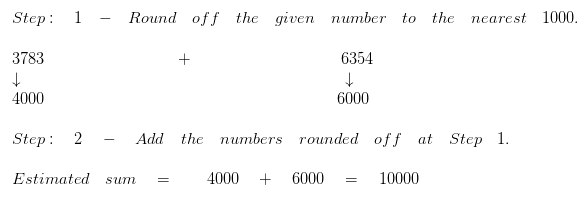## Estimate the Difference

Question 1

Find the estimated difference:
58 – 24

Explanation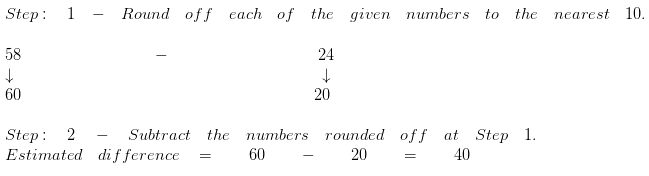Question 2

Find the estimated difference:
512 – 398

Explanation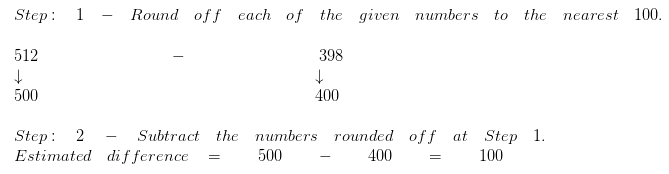Question 3

Find the estimated difference:
7839 – 4356

Explanation